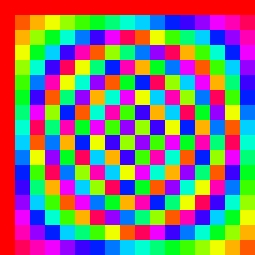# Cayley TablesA Cayley Table is a multiplication table for a group. This sounds simple however some interesting patterns and things come from this.

For example take the integers modulo 3, denoted as $$\Bbb Z_3$$. Let's define multiplication in the usual sense. You will find that this forms a group (you can check this).

Now writing the elements of the group out in Lexicographical order we can form a table.

 $\times$ $0$ $1$ $2$ $0$ $1$ $2$

Now we simply fill it in by applying the multiplication to the corresponding elements in the rows and columns in the table. You should have:

 $\times$ $0$ $1$ $2$ $0$ $0$ $0$ $0$ $1$ $0$ $1$ $2$ $2$ $0$ $2$ $1$

Now if I colour the elements lexicographically we can see a pattern emerge (more clearly).

 $\times$ $\color{#D61F06}0$ $\color{#20A900}1$ $\color{#3D99F6}2$ $\color{#D61F06}0$ $\color{#D61F06}0$ $\color{#D61F06}0$ $\color{#D61F06}0$ $\color{#20A900}1$ $\color{#D61F06}0$ $\color{#20A900}1$ $\color{#3D99F6}2$ $\color{#3D99F6}2$ $\color{#D61F06}0$ $\color{#3D99F6}2$ $\color{#20A900}1$

We can go further and assume lexicographical order (of some sort) and remove the reference rows and columns.

 $\color{#D61F06}0$ $\color{#D61F06}0$ $\color{#D61F06}0$ $\color{#D61F06}0$ $\color{#20A900}1$ $\color{#3D99F6}2$ $\color{#D61F06}0$ $\color{#3D99F6}2$ $\color{#20A900}1$

The interesting thing is, is that we can do this for any group and reveal some of it's symmetries and patterns.

Over the next few days I shall be releasing the coloured Cayley tables for some groups that I have generated. We shall start of with simpler groups and eventually get more complicated. But first:

The Image at the top of the post is the Cayley Table for $\Bbb Z_{17}$Note by A Former Brilliant Member
7 years, 1 month ago

This discussion board is a place to discuss our Daily Challenges and the math and science related to those challenges. Explanations are more than just a solution — they should explain the steps and thinking strategies that you used to obtain the solution. Comments should further the discussion of math and science.

When posting on Brilliant:

• Use the emojis to react to an explanation, whether you're congratulating a job well done , or just really confused .
• Ask specific questions about the challenge or the steps in somebody's explanation. Well-posed questions can add a lot to the discussion, but posting "I don't understand!" doesn't help anyone.
• Try to contribute something new to the discussion, whether it is an extension, generalization or other idea related to the challenge.

MarkdownAppears as
*italics* or _italics_ italics
**bold** or __bold__ bold
- bulleted- list
• bulleted
• list
1. numbered2. list
1. numbered
2. list
Note: you must add a full line of space before and after lists for them to show up correctly
paragraph 1paragraph 2

paragraph 1

paragraph 2

[example link](https://brilliant.org)example link
> This is a quote
This is a quote
    # I indented these lines
# 4 spaces, and now they show
# up as a code block.

print "hello world"
# I indented these lines
# 4 spaces, and now they show
# up as a code block.

print "hello world"
MathAppears as
Remember to wrap math in $$ ... $$ or $ ... $ to ensure proper formatting.
2 \times 3 $2 \times 3$
2^{34} $2^{34}$
a_{i-1} $a_{i-1}$
\frac{2}{3} $\frac{2}{3}$
\sqrt{2} $\sqrt{2}$
\sum_{i=1}^3 $\sum_{i=1}^3$
\sin \theta $\sin \theta$
\boxed{123} $\boxed{123}$